Dr. S. R. Lasker Library Online Catalogue

Normal view

# The R book / Michael J Crawley

Material type:TextLanguage: English Publication details: 2013. Description: xxiv, 1051 p. : ill. ; 25 cmISBN: 9780470973929DDC classification: 519.502855133 Online resources: Ebook Fulltext | Ebook Fulltext
Contents:
TOC Preface -- 1. Getting Started -- 2. Essentials of the R Language -- 3. Data Input -- 4. Dataframes -- 5. Graphics -- 6 Tables -- 7. Mathematics -- 8. Classical Tests -- 9. Statistical Modelling -- 10. Regression -- 11. Analysis of Variance -- 12. Analysis of Covariance -- 13. Generalized Linear Models -- 14. Count Data -- 15. Count Data in Tables -- 16. Proportion Data -- 17. Binary Response Variables -- 18. Generalized Additive Models -- 19. Mixed-Effects Models -- 20. Non-linear Regression -- 21. Meta-analysis -- 22. Bayesian statistics -- 23. Tree Models -- 24. Time Series Analysis -- 25. Multivariate Statistics -- 26. Spatial Statistics -- 27. Survival Analysis -- 28. Simulation Models -- 29. Changing the Look of Graphics.
Summary: "Hugely successful and popular text presenting an extensive and comprehensive guide for all R users The R language is recognized as one of the most powerful and flexible statistical software packages, enabling users to apply many statistical techniques that would be impossible without such software to help implement such large data sets. R has become an essential tool for understanding and carrying out research.This
Tags from this library: No tags from this library for this title.
Star ratingsAverage rating: 0.0 (0 votes)
Holdings
Item type Current library Collection Call number Copy number Status Date due Barcode Item holdsE-Book
E-book
Non-fiction 519.502855133 CRR 2013 (Browse shelf(Opens below)) Not for loanText
Reserve Section
Non-fiction 519.502855133 CRR 2013 (Browse shelf(Opens below)) C-1 Not For Loan 27149Text
Circulation Section
Non-fiction 519.502855133 CRR 2013 (Browse shelf(Opens below)) C-2 Available 27150
Total holds: 0
##### Browsing EWU Library shelves, Shelving location: E-book Close shelf browser (Hides shelf browser)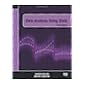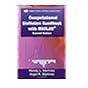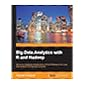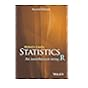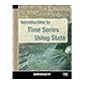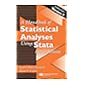519.50285 KOD 2012 Data analysis using stata / 519.50285 MAC Computational statistics handbook with MATLAB / 519.50285 PRB 2013 Big data analytics with R and Hadoop / 519.502855133 CRR 2013 The R book / 519.502855133 CRS 2015 Statistics : 519.50285536 BEI 2013 Introduction to time series using Stata / 519.50285536 HEH 2007 A handbook of statistical analyses using Stata /

Includes bibliographical references and index.

TOC Preface --
1. Getting Started --
2. Essentials of the R Language --
3. Data Input --
4. Dataframes --
5. Graphics --
6 Tables --
7. Mathematics --
8. Classical Tests --
9. Statistical Modelling --
10. Regression --
11. Analysis of Variance --
12. Analysis of Covariance --
13. Generalized Linear Models --
14. Count Data --
15. Count Data in Tables --
16. Proportion Data --
17. Binary Response Variables --
19. Mixed-Effects Models --
20. Non-linear Regression --
21. Meta-analysis --
22. Bayesian statistics --
23. Tree Models --
24. Time Series Analysis --
25. Multivariate Statistics --
26. Spatial Statistics --
27. Survival Analysis --
28. Simulation Models --
29. Changing the Look of Graphics.

"Hugely successful and popular text presenting an extensive and comprehensive guide for all R users The R language is recognized as one of the most powerful and flexible statistical software packages, enabling users to apply many statistical techniques that would be impossible without such software to help implement such large data sets. R has become an essential tool for understanding and carrying out research.This

AS

Saifun Momota

There are no comments on this title.